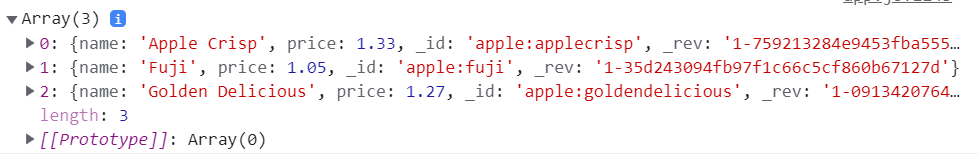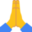# B4J Library[BANanoRelax] 1. Perform CRUD Offline, 2. Sync to Cloud and 3. Relax

Ola

The BANanoRelax library allows one to work offline, sync the crud data to cloud storage and basically relax. For more details, see this thread.

Getting Started

1. Include the attached BANanoRelax Class
2. In AppStart, include the following lines

B4X:
``````BANano.header.AddJavascriptFile("pouchdb.min.js")

See Examples on Main (output is on console log)

For more examples, see this post:

BANanoRelax

• Operators
B4X:
``````Public const OP_EQ As String = "\$eq"
Public const OP_LTE As String = "\$lte"
Public const OP_LT As String = "\$lt"
Public const OP_GT As String = "\$gt"
Public const OP_GTE As String = "\$gte"
Public const OP_EXISTS As String = "\$exists"
Public const OP_NE As String = "\$ne"
Public const OP_IN As String = "\$in"
Public const OP_NOT_IN As String = "\$nin"
Public const OP_SIZE As String = "\$size"
Public const OP_MOD As String = "\$mod"
Public const OP_REGEX As String = "\$regex"
Public const OP_ELEM_MATCH As String = "\$elemMatch"
Public const OP_TYPEOF As String = "\$type"
Public const OP_ALL As String = "\$all"``````
• setHost (vHost As String)
set the remote host for synch
B4X:
``db.Host = "127.0.0.1"``
• setPort (vPort As String)
set the remote port
B4X:
``db.Port = "5984"``
B4X:
``db.UserName = "xxx"``
B4X:
``db.Password = "xxx"``
• INITIALIZE (Module As Object, name As String, auto As Boolean) As BANanoRelax
B4X:
``````'open the database for processing, true ensures BANano.GUIDs are generated
'name - name of database
'auto - own _id / generated by database, set false for own

Dim db As BANanoRelax
db.Initialize(Me, "mydb", true)
db.WORK_OFFLINE``````
• WORK_OFFLINE As BANanoRelax
this creates an offline IndexDB database
• WORK_ONLINE
connects directly with the online DB for your CRUD (does not sync, just opens an online connection)
• SYNC (live As Boolean, retry As Boolean)
sync with remote DB
B4X:
``````db.Host = "localhost"
db.Port = "5984"
'live - true (continuously)
'retry - when true retries if offline
db.Sync(True, True)

'the callback for the sync errors
Sub mydb_syncerror()
End Sub``````
• WATCH_CHANGES (sinceWhen As String, live As Boolean, includedocs As Boolean) As BANanoRelax
B4X:
``````'watch when changes take place
db.WatchChanges("now", True, True)

'changes callback
Sub db_change(change As Map)
End Sub

'complete callback
Sub db_complete(info As Map)
End Sub

'error callback
Sub db_error(err As Map)
End Sub``````
• INSERT (nrec As Map) As BANanoRelax
'the callback for the insert
• B4X:
``````Dim person As Map = CreateMap()
person.Put("_id", "1")
person.Put("firstname", "Anele")
person.Put("lastname", "Mbanga")
[*]db.INSERT(person)
[*]

[*]Sub mydb_insert(Err As RelaxErr, Rec As RelaxRec)
End Sub
[/code'
[*]PutWait (nrec As Map) As Map
'same as insert but uses wait
[code]
Dim person As Map = CreateMap()
person.Put("_id", "1")
person.Put("firstname", "Anele")
person.Put("lastname", "Mbanga")
Dim res As Map = BANano.Await(db.PutWait(person))``````
• GetAllWait (desc As Boolean) As List
'works the same as SELECT_ALL but uses wait
B4X:
``````Dim recs As List = BANano.Await(db.GetAllWait(True))
Log(recs)``````
• GetWait (docID As String) As Map
same as read by uses wait
B4X:
``Dim rg As Map = BANano.Await(db.GetWait("1"))``
• RemoveWait (docID As String) As Map
same as delete but uses wait
B4X:
``Dim rd As Map = BANano.Await(db.RemoveWait("1"))``
• UpdateWait (docID As String, nrec As Map, force As Boolean) As Map
same as update but uses wait
B4X:
``````rg.Put("firstname", "Usibabale")
Dim ru As Map = BANano.Await(db.UpdateWait("1", rg, True))``````
• READ (docID As String) As BANanoRelax

B4X:
``````'read a record from a table using the id

'the callback for the insert
Sub mydb_read(Err As RelaxErr, Doc As Map)
End Sub``````
• DELETE (nrec As Map) As BANanoRelax
B4X:
``````'delete the database record (use record with id and rev)
db.DELETE(rec)

'the callback for the delete
Sub mydb_delete(Err As RelaxErr, Rec As RelaxRec)
End Sub``````
• UPDATE (nrec As Map, force As Boolean) As BANanoRelax
B4X:
``````'update the database record (use record with id and rev)
db.UPDATE(rec)

'the callback for the update
Sub mydb_update(Err As RelaxErr, Rec As RelaxRec)
End Sub``````
• INSERT_BULK (nrecs As List) As BANanoRelax
B4X:
``````'insert bulk records, _id can be BANano.GenerateUUID
Dim lst As List
lst.Initialize
lst.Add(CreateMap("title" : "Lisa Says", "_id": "doc1"))
lst.Add(CreateMap("title" : "Space Oddity", "_id": "doc2"))
db.INSERT_BULK(lst)

'the callback for the insert
Sub mydb_insert(Err As RelaxErr, Rec As RelaxRec)
End Sub``````
• InsertBulkWait (recs As List) As List
works like insert bulk but using Wait
B4X:
``````'insert bulk records, _id can be BANano.GenerateUUID
Dim lst As List
lst.Initialize
lst.Add(CreateMap("title" : "Lisa Says", "_id": "doc1"))
lst.Add(CreateMap("title" : "Space Oddity", "_id": "doc2"))
Dim res As Map = BANano.Await(db.InsertBulkWait(lst))``````
• SELECT_ALL (desc As Boolean) As BANanoRelax
B4X:
``````'select all records from the data-base
'desc of True means show new-west on top sorted by ID, useful for datenow ids
db.SELECT_ALL(True)

'the callback for the select all
Sub mydb_selectall(Err As RelaxErr, Documents As List)
For each rec As Map in Documents
Next
End Sub``````
• CREATE_INDEX (xFields As List) As BANanoRelax
B4X:
``````'create an index based on fields
db.CREATE_INDEX(Array("firstname", "lastname"))

'the callback for the select all
Sub mydb_createindex(Err As RelaxErr, Documents As List)
For each rec As Map in Documents
Next
End Sub``````
• CreateIndexWait (xFields As List) As Map
creates an index before a search is done
B4X:
``````Dim res As Map = BANano.Await(db.CreateIndexWait(Array("firstname", "lastname")))
Log(res)``````
• SelectWhereWait As List
B4X:
``````'specify the fields and the values to find, this will create an index on the SortBy fields
'* means all fields else specify the field names to return
'the operators indicates the condition that field should meet
'SELECT * FROM db WHERE firstname = 'Usibabale' ORDER BY _id desc
db.NEW_QUERY
Dim docs As List = db.SelectWhereWait()
for each rec As Map in docs
next``````
• SELECT_WHERE As BANanoRelax
B4X:
``````'SELECT * FROM db WHERE firstname = 'Usibabale' ORDER BY _id desc
'specify the fields and the values to find, this will create an index based on the sort by fields
'* means all fields else specify the field names to return
db.NEW_QUERY
db.SELECT_WHERE

'the callback for the find all
Sub mydb_selectwhere(docs As List)
For each rec As Map in docs
Next
End Sub``````
• NEW_QUERY As BANanoRelax
clear where clause
B4X:
``````'create a new query
db.NEW_QUERY``````
• ADD_FIELDS (flds As List) As BANanoRelax
'set the fields for the select

• ADD_ORDER_BY (flds As List) As BANanoRelax
set the sort order
• ADD_WHERE (fld As String, operator As String, value As Object) As BANanoRelax
B4X:
``db.ADD_WHERE("firstname", db.EQ, "Usibabale")``
• CLOSE
close the database connection

Last edited:
•Gabino A. de la Gala and LJG

#### Mashiane

##### Expert
Longtime User
How to Query?

1. We initialize our database for CRUD

B4X:
``````Dim games As BANanoRelax

games.INITIALIZE(Me, "games", False)
games.Host = "127.0.0.1"
games.Port = "5984"
games.WORK_OFFLINE``````

2. We will create a list of records that we will add to our IndexedDB database.

B4X:
``````Sub BulkGames As List
Dim lst As List
lst.Initialize
lst.Add(CreateMap("name": "Donkey Kong", "series": "Mario", "debut": 1981, "_id": "dk"))
lst.Add(CreateMap("name": "Captain Falcon", "series": "F-Zero", "debut": 1990, "_id": "falcon"))
lst.Add(CreateMap("name": "Fox", "series": "Star Fox", "debut": 1993, "_id": "fox"))
lst.Add(CreateMap("name": "Kirby", "series": "Kirby", "debut": 1992, "_id": "kirby"))
lst.Add(CreateMap("name": "Luigi", "series": "Mario", "debut": 1983, "_id": "luigi"))
lst.Add(CreateMap("name": "Mario", "series": "Mario", "debut": 1981, "_id": "mario"))
lst.Add(CreateMap("name": "Ness", "series": "Earthbound", "debut": 1994, "_id": "ness"))
lst.Add(CreateMap("name": "Pikachu", "series": "Pokemon", "debut": 1996, "_id": "pikachu"))
lst.Add(CreateMap("name": "Jigglypuff", "series": "Pokemon", "debut": 1996, "_id": "puff"))
lst.Add(CreateMap("name": "Samus", "series": "Metroid", "debut": 1986, "_id": "samus"))
lst.Add(CreateMap("name": "Yoshi", "series": "Mario", "debut": 1990, "_id": "yoshi"))
Return lst
End Sub``````

3. We add our games to the DB

B4X:
``````'add most games
Dim lst As List = BulkGames
Dim res As List = BANano.Await(games.InsertBulkWait(lst))
Log(res)``````

4. We can sync our offline db to online, you can then view it with the Faux GUI

B4X:
``games.SYNC(True, True)``

5. Lets run some queries

B4X:
``````'select * from games
Dim res As List = BANano.Await(games.SelectWhereWait)
Log(res)``````

B4X:
``````'select * from games where _id >= 'dk' order by _id
Dim res As List = BANano.Await(games.SelectWhereWait)
Log(res)``````

B4X:
``````'select * from games order by name"
Dim res As List = BANano.Await(games.SelectWhereWait)
Log(res)``````

B4X:
``````'select * from games where debut >= 1990
Dim res As List = BANano.Await(games.SelectWhereWait)
Log(res)``````

B4X:
``````'select * from games where series = 'Mario' order by series desc, debut desc
Dim res As List = BANano.Await(games.SelectWhereWait)
Log(res)``````

B4X:
``````'select _id, debut from games order by debut"
Dim res As List = BANano.Await(games.SelectWhereWait)
Log(res)``````

B4X:
``````'select * from games where series in ('Mario','Zelda') order by _id
games.NEW_QUERY
Dim res As List = BANano.Await(games.SelectWhereWait)``````

B4X:
``````'select * from games where series = 'Mario' and debut > 1981 order by name desc)
games.NEW_QUERY
Dim res As List = BANano.Await(games.SelectWhereWait)
Log(res)``````

Last edited:
•LJG

#### Mashiane

##### Expert
Longtime User
How to CRUD?

NB: To update & delete records you always need the id and _rev of the document.

1. CREATE

B4X:
``````Dim person As Map = CreateMap()
person.Put("_id", "1")
person.Put("firstname", "Anele")
person.Put("lastname", "Mbanga")

Dim res As Map = BANano.Await(db.PutWait(person))
Log(res)``````

B4X:
``````Dim rg As Map = BANano.Await(db.GetWait("1"))
Log(rg)``````

3. UPDATE (we are using the previsouly read record, we need the _id and _rev to update a record

B4X:
``````rg.Put("firstname", "Usibabale")
Dim ru As Map = BANano.Await(db.UpdateWait("1", rg, True))
Log(ru)``````

4. DELETE

B4X:
``````Dim rd As Map = BANano.Await(db.RemoveWait("1"))
Log(rd)``````

5. SELECT ALL

B4X:
``````Dim recs As List = BANano.Await(db.GetAllWait(True))
Log(recs)``````

•behnam_tr and LJG

#### Mashiane

##### Expert
Longtime User
Handling Ranges

By default the _id field is always indexed and you dont have to use .CreateIndex for it.

Whenever you use .ADD_WHERE and .ADD_SORT_BY - an index is created based on the fields of your query (if it does not exists)

Ranges are kinda interesting...

B4X:
``````Sub BulkFruit As List
Dim lst As List
lst.Initialize
lst.Add(CreateMap("_id": "apple:fuji", "name": "Fuji", "price": 1.05))
lst.Add(CreateMap("_id": "apple:applecrisp", "name": "Apple Crisp", "price": 1.33))
lst.Add(CreateMap("_id": "pear:bosc", "name": "Bosc", "price": 1.95))
lst.Add(CreateMap("_id": "apple:goldendelicious", "name": "Golden Delicious", "price": 1.27))
lst.Add(CreateMap("_id": "pear:bartlett", "name": "Bartlett", "price": 1.02))
Return lst
End Sub``````

For example, we are adding some fruits here, and we decide to prefix the id with the kind of fruit that is. With this we dont have to create another index.

We add these to our database:

B4X:
``````Dim fruit As List = BulkFruit
Dim res As List = BANano.Await(games.InsertBulkWait(fruit))
Log(res)``````

Now we want to only extract apples.

B4X:
``````Dim apples As List = BANano.Await(games.GetRangeWait("apple:", True))
Log(apples)``````

Output:••joulongleu, behnam_tr and LJG